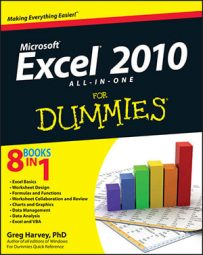##### Excel 2010 All-in-One For DummiesYou activate Excel 2010 hot keys by pressing the Alt key before you type the mnemonic letter for a particular task. Hot key sequences for the most common formula-related commands in Excel 2010 begin with the sequence Alt+M because the M in forMulas was the only mnemonic key still available (F is assigned to the File menu commands). After you know to press Alt+M to access Formula tab commands, it’s pretty easy sailing.

Hot Key Excel Ribbon Command Function
Alt+MF Formulas→Insert Function Opens the Insert Function dialog box (same as clicking the Insert Function button on the Formula bar
Alt+MUS Formulas→AutoSum→Sum Selects the occupied range above the cell cursor and inserts SUM formula to total the range
Alt+MUA Formulas→AutoSum→Average Selects the occupied range above the cell cursor and inserts AVERAGE formula to calculate the average of total in the range
Alt+MUC Formulas→AutoSum→Count Numbers Selects the occupied range above the cell cursor and inserts COUNT formula to count the number of values in the range
Alt+MI Formulas→Financial Opens a drop-down menu listing all Financial functions — click name to insert function into current cell
Alt+ME Formulas→Date & Time Opens a drop-down menu listing all Date and Time functions — click name to insert function into current cell
Alt+MN Formulas→Name Manager Opens Name Manager dialog box showing all range names in workbook where you can add, edit, and delete names
Alt+MMD Formulas→Define Name Opens New Name dialog box where you can assign a name to the cell selection or define a new constant
Alt+MS Formulas→Use in Formula Displays drop-down menu with range names in workbook that you can insert into current formula by clicking
Alt+MC Formulas→Create from Selection Opens Create Names from Selection dialog box where you indicate which rows and columns to use in naming cell selection
Alt+MH Formulas→Show Formulas (Ctrl+`) Displays and then hides all formulas in cells of the worksheet
Alt+MXA Formulas→Options→Automatic Turns automatic recalculation back on
Alt+MXE Formulas→Options→Automatic Except for Data Tables Turns automatic recalculation back on for all parts of the worksheet except for ranges with Data Tables
Alt+MXM Formulas→Options→Manual Turns on manual recalculation
Alt+MB Formulas→Calculate Now (F9) Recalculates formulas throughout the entire workbook when manual recalculation is turned on
Alt+MJ Formulas→Calculate Sheet (Shift+F9) Recalculates formulas in the current worksheet when manual recalculation is turned on